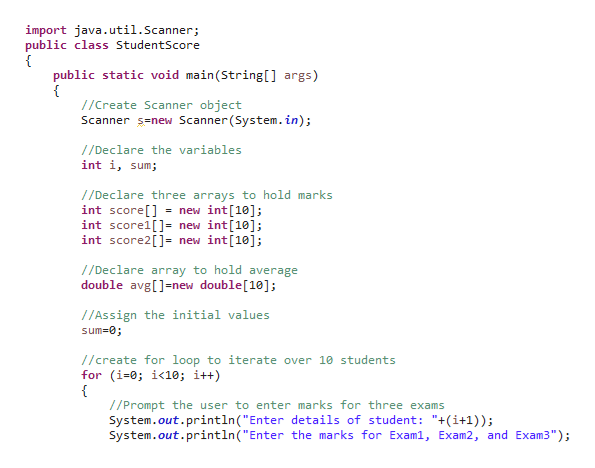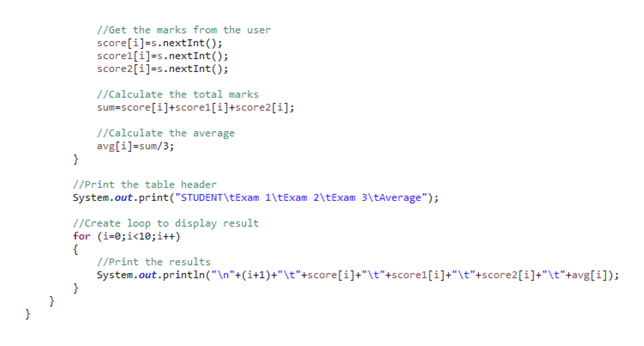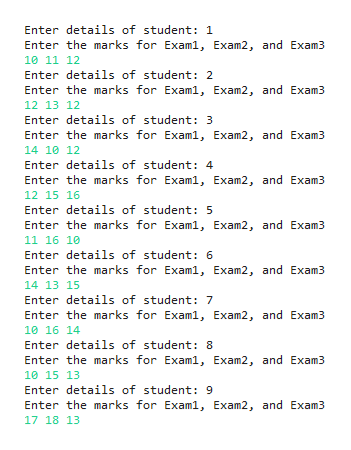# using java a class of 10 students that will take 3 exams. use 3 arrays to hold the scores for each student and another to hold the average of the 3 exams for each student. print a table with the scores and average

Question
31 views

using java a class of 10 students that will take 3 exams. use 3 arrays to hold the scores for each student and another to hold the average of the 3 exams for each student. print a table with the scores and average

check_circle

Step 1

A Java program for the given criteria as follows,

File name: “StudentScore.java”

import java.util.Scanner;

public class StudentScore

{

public static void main(String[] args)

{

//Create Scanner object

Scanner s=new Scanner(System.in);

//Declare the variables

int i, sum;

//Declare three arrays to hold marks

int score[] = new int;

int score1[]= new int;

int score2[]= new int;

//Declare array to hold average

double avg[]=new double;

//Assign the initial values

sum=0;

//create for loop to iterate over 10 students

for (i=0; i<10; i++)

{

//Prompt the user to enter marks for three exams

System.out.println("Enter details of student: "+(i+1));

System.out.println("Enter the marks for Exam1, Exam2, and Exam3");

//Get the marks from the user

score[i]=s.nextInt();

score1[i]=s.nextInt();

score2[i]=s.nextInt();

//Calculate the total marks

sum=score[i]+score1[i]+score2[i];

//Calculate the average

avg[i]=sum/3;

}

System.out.print("STUDENT\tExam 1\tExam 2\tExam 3\tAverage");

//Create loop to display result

for (i=0;i<10;i++)

{

//Print the results

System.out.println("\n"+(i+1)+"\t"+score[i]+"\t"+score1[i]+"\t"+score2[i]+"\t"+avg[i]);

}

}

}

Screenshot of program #1:help_outlineImage Transcriptioncloseimport java.util.Scanner; public class StudentScore public static void main(String [] args) { //Create Scanner object Scanner s-new Scanner(System.in) //Declare the variables int i, sum; //Declare three arrays to hold marks int score[] int score1[]= new int int score2[] new int; = new int ]; //Declare array to hold average double avg[new double; //Assign the initial values sum-0; //create for loop to iterate over 10 students for (i-0; i<10; i++ { //Prompt the user to enter marks for three exams System.out.println ( " Enter details of student: "+(i+1)); System.out.println (" Enter the marks for Exam1, Exam2, and Exam3"); fullscreen
Step 2

Screenshot of program #2:help_outlineImage Transcriptionclose/Get the marks from the user score[il-s.nextInt (); scorei[i-s.nextInt ( ); score2[i]-s.nextInt (); //Calculate the total marks sum-score[i]+score1 [i] +score2 [ i] ; 1/Calculate the average avg[i-sum/3; } //Print the table header System.out.print("STUDENT\t Exam 1\tExam 2\tExam 3\tAverage"); 1/Create loop to display result for (i-0;i<10; i++ //Print the results System.out.println ("\n"+(i+1) #"\t"+score [i] +" \t"+score1 [ i] +" \t"+score2 [i] +"\t"+avg [i] ) fullscreen
Step 3

Screenshot of output #1...help_outlineImage TranscriptioncloseEnter details of student: 1 Enter the marks for Exam1, Exam2, and Exam3 10 11 12 Enter details of student: 2 Enter the marks for Examl, Exam2, and Exam3 12 13 12 Enter details of student: 3 Enter the marks for Examl, Exam2, and Exam3 14 10 12 Enter details of student: 4 Enter the marks for Exam1, Exam2, and Exam3 12 1516 Enter details of student: 5 Enter the marks for Exam1, Exam2, and Exam3 11 16 10 Enter details of student: 6 Enter the marks for Examl, Exam2, and Exam3 14 13 15 Enter details of student: 7 Enter the marks for Exam1, Exam2, and Exam3 10 16 14 Enter details of student: 8 Enter the marks for Examl, Exam2, and Exam3 10 15 13 Enter details of student: 9 Enter the marks for Examl, Exam2, and Exam3 17 18 13 fullscreen

### Want to see the full answer?

See Solution

#### Want to see this answer and more?

Solutions are written by subject experts who are available 24/7. Questions are typically answered within 1 hour.*

See Solution
*Response times may vary by subject and question.
Tagged in
EngineeringComputer Science

### Java